# Thinking [email protected] #4[email protected] is a series of questions that we, as tutors feel that are useful in helping students think and improve their understanding.

Thinking [email protected] is curated by KS. More of him can be found here.

This is a question from 1993 Paper 1.

The positive integers, starting at 1, are grouped into sets containing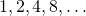integers, as indicated below, so that the number of integers in each set after the first is twice the number of integers in the previous set.(i) Write down the expressions, in terms offor

(a) the number of integers in theset,

(b) the first integer in theset,

(c) the last integer in theset.

(ii) Given that the integeroccurs in theset, find the integer value of.

(iii) The sum of all the integers in the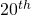set is denoted by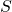, and the sum of all the integers in all of the firstsets is denoted by. Show thatmay be expressed as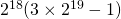.

Hence, evaluate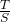, correct to 4 decimal places.

Not readable? Change text.